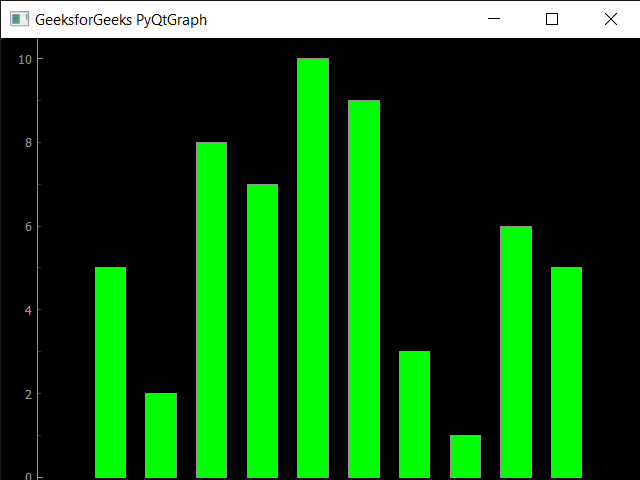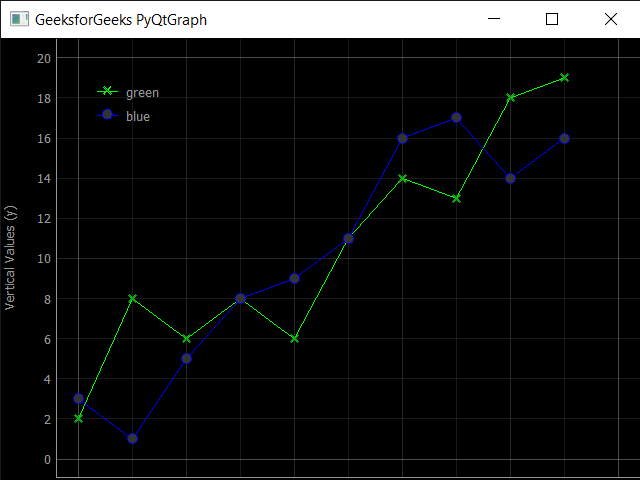Introduction to PyQtGraph Module in Python

• Difficulty Level : Medium
• Last Updated : 01 Jun, 2021

PyQtGraph is a graphics and user interface library for Python that provides functionality commonly required in designing and science applications. Its primary goals are to provide fast, interactive graphics for displaying data (plots, video, etc.) and second is to provide tools to aid in rapid application development (for example, property trees such as used in Qt Designer).
PyQtGraph makes heavy use of the Qt GUI platform (via PyQt or PySide) for its high-performance graphics and numpy for heavy number crunching. In particular, pyqtgraph uses Qt’s GraphicsView framework which is a highly capable graphics system on its own, this brings optimized and simplified primitives to this framework to allow data visualization with minimal effort.
In order to install the PyQtGraph we use the command given below

pip install pyqtgraph

Requirements: PyQtGraph can run on any platform which supports the following packages:
1. Python 2.7 and 3+
2. PyQt 4.8+ or PySide
3. NumPy
4. python-opengl bindings are required for 3D graphics
Example : In this example we will create a simple bar graph using PyQtGraph module in python

Python3

 # importing pyqtgraph as pgimport pyqtgraph as pg # importing QtCore and QtGui from the pyqtgraph modulefrom pyqtgraph.Qt import QtCore, QtGui # importing numpy as npimport numpy as np # creating a pyqtgraph plot windowwindow = pg.plot() # titletitle = "GeeksforGeeks PyQtGraph" # setting window titlewindow.setWindowTitle(title) # create list for y-axisy1 = [5, 5, 7, 10, 3, 8, 9, 1, 6, 2] # create horizontal list i.e x-axisx = [1, 10, 4, 5, 7, 3, 6, 8, 9, 2] # create pyqt5graph bar graph item# with bar colors = greenbargraph1 = pg.BarGraphItem(x = x, height = y1, width = 0.6, brush ='g') # adding bargraph item to the windowwindow.addItem(bargraph1) # main methodif __name__ == '__main__':         # importing system    import sys         # Start Qt event loop unless running in interactive mode or using    if (sys.flags.interactive != 1) or not hasattr(QtCore, 'PYQT_VERSION'):        QtGui.QApplication.instance().exec_()

Output :Example : In this we will plot two lines together in same graph, below is the implementation

Python3

 # importing pyqtgraph as pgimport pyqtgraph as pg # importing QtCore and QtGui from the pyqtgraph modulefrom pyqtgraph.Qt import QtCore, QtGui # importing numpy as npimport numpy as np # define the datatitle = "GeeksforGeeks PyQtGraph" # y values to plot by line 1y = [2, 8, 6, 8, 6, 11, 14, 13, 18, 19] # y values to plot by line 2y2 = [3, 1, 5, 8, 9, 11, 16, 17, 14, 16]x = range(0, 10) # create plot objectplt = pg.plot() # showing x and y gridsplt.showGrid(x = True, y = True) # adding legendplt.addLegend() # set properties of the label for y axisplt.setLabel('left', 'Vertical Values', units ='y') # set properties of the label for x axisplt.setLabel('bottom', 'Horizontal Values', units ='s') # setting horizontal rangeplt.setXRange(0, 10) # setting vertical rangeplt.setYRange(0, 20) # setting window titleplt.setWindowTitle(title) # ploting line in green colorline1 = plt.plot(x, y, pen ='g', symbol ='x', symbolPen ='g', symbolBrush = 0.2, name ='green') # ploting line2 with blue colorline2 = plt.plot(x, y2, pen ='b', symbol ='o', symbolPen ='b', symbolBrush = 0.2, name ='blue')   # main methodif __name__ == '__main__':         # importing system    import sys         # Start Qt event loop unless running in interactive mode or using    if (sys.flags.interactive != 1) or not hasattr(QtCore, 'PYQT_VERSION'):        QtGui.QApplication.instance().exec_()

Output :Things PyQtGraph can perform :
1. Basic data visualization like images, line and scatter plots
2. Fast enough to plot realtime update of video / data
3. Interactive scaling/panning, averaging, FFTs, SVG/PNG export
4. Widgets for marking & selecting plot regions
5. Automatic slicing of multi-dimensional image data
6. Framework for building customized image region-of-interest widgets
7. Docking system that replaces/complements Qt’s dock system to allow more complex (and more predictable) docking arrangements
8. ParameterTree widget for rapid prototyping of dynamic interfaces

My Personal Notes arrow_drop_up